• ## 特征分解

千次阅读 2019-04-16 13:27:00
特征分解（eigendecomposition）是使用最广的矩阵分解之一，即我们将矩阵分解成一组特征向量和特征值。 方阵 A 的 特征向量（eigenvector）是指与 A 相乘后相当于对该向量进行缩放的非零向量 v： 标量 λ 被称为...


特征分解（eigendecomposition）是使用最广的矩阵分解之一，即我们将矩阵分解成一组特征向量和特征值。
方阵 A 的 特征向量（eigenvector）是指与 A 相乘后相当于对该向量进行缩放的非零向量 v：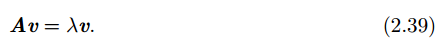标量 λ 被称为这个特征向量对应的 特征值（eigenvalue）。（类似地，我们也可以 定义 左特征向量（left eigenvector） v⊤A = λv⊤，但是通常我们更关注 右特征向量 （right eigenvector））。
如果 v 是 A 的特征向量，那么任何缩放后的向量 sv (s ∈ R， s ≠ 0) 也是 A 的 特征向量。此外， sv 和 v 有相同的特征值。基于这个原因，通常我们只考虑单位特征向量。
假设矩阵 A 有 n 个线性无关的特征向量 {v(1),...,v(n)}，对应着特征值{λ1, ..., λn}。我们将特征向量连接成一个矩阵，使得每一列是一个特征向量： V =  [v(1),...,v(n)]. 类似地，我们也可以将特征值连接成一个向量 λ = [λ1, ..., λn]⊤。 因此 A 的 特征分解（eigendecomposition）可以记作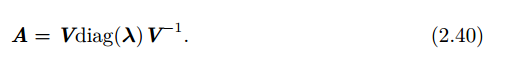* diag(λ）向量λ在n*n的方针的对角线上。

正交矩阵的转置和逆矩阵相等设A是对称矩阵A^T = AA^-1 = (A^T)^-1 = (A^-1)^T (即A的逆也是对称矩阵)

我们已经看到了构建具有特定特征值和特征向量的矩阵，能够使我们在目标方向上延伸空间。然而，我们也常常希望将矩阵分解（decompose）成特征值和特征向量。这样可以帮助我们分析矩阵的特定性质。不是每一个矩阵都可以分解成特征值和特征向量。在某些情况下，特征分解存在，但是会涉及复数而非实数。在深度学习中，我们通常只需要分解一类有简单分解的矩阵。具体来讲，每个实对称矩阵都可以分解成实特征向量和实特征值：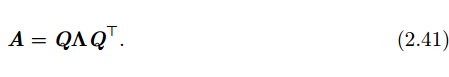其中 Q 是 A 的特征向量组成的正交矩阵， Λ 是对角矩阵。特征值 Λi,i 对应的特征向量是矩阵 Q 的第 i 列，记作 Q:,i。因为 Q 是正交矩阵，我们可以将 A 看作沿方 向 v(i) 延展 λi 倍的空间。如图 2.3 所示的例子。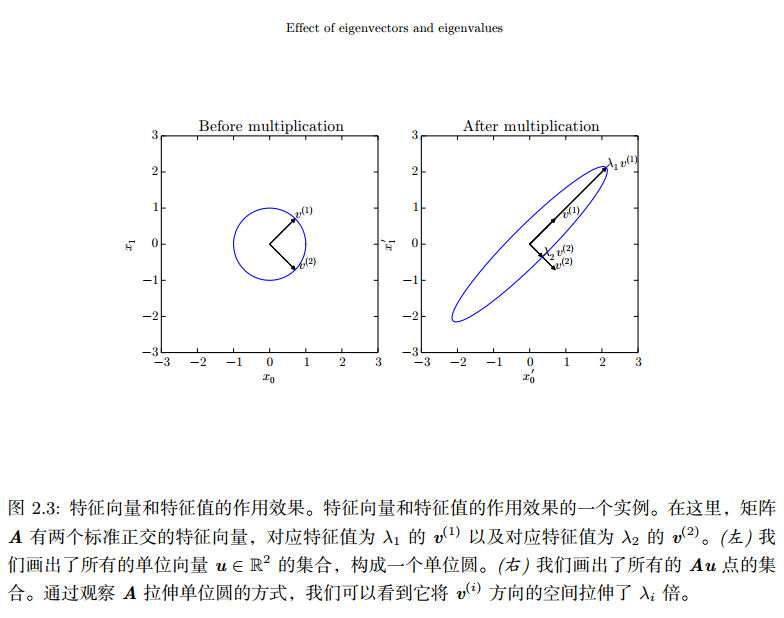虽然任意一个实对称矩阵 A 都有特征分解，但是特征分解可能并不唯一。如果两个或多个特征向量拥有相同的特征值，那么在由这些特征向量产生的生成子空间中，任意一组正交向量都是该特征值对应的特征向量。因此，我们可以等价地从这些特征向量中构成 Q 作为替代。按照惯例，我们通常按降序排列 Λ 的元素。在该约定下，特征分解唯一当且仅当所有的特征值都是唯一的。
矩阵的特征分解给了我们很多关于矩阵的有用信息。矩阵是奇异的当且仅当含 有零特征值。实对称矩阵的特征分解也可以用于优化二次方程 f(x) = x⊤Ax，其中 限制 ∥x∥2 = 1。当 x 等于 A 的某个特征向量时， f 将返回对应的特征值。在限制条 件下，函数 f 的最大值是最大特征值，最小值是最小特征值。
所有特征值都是正数的矩阵被称为 正定（positive definite）；所有特征值都是非负数的矩阵被称为 半正定（positive semidefinite）。同样地，所有特征值都是负数的矩阵被称为 负定（negative definite）；所有特征值都是非正数的矩阵被称为 半负定 （negative semidefinite）。 半正定矩阵受到关注是因为它们保证 x⊤Ax ≥ 0。此外， 正定矩阵还保证 x⊤Ax = 0 → x = 0。

正交矩阵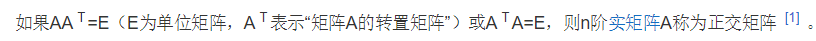正交化
如果是实对称矩阵，那么它的不同特征值的特征向量必然正交。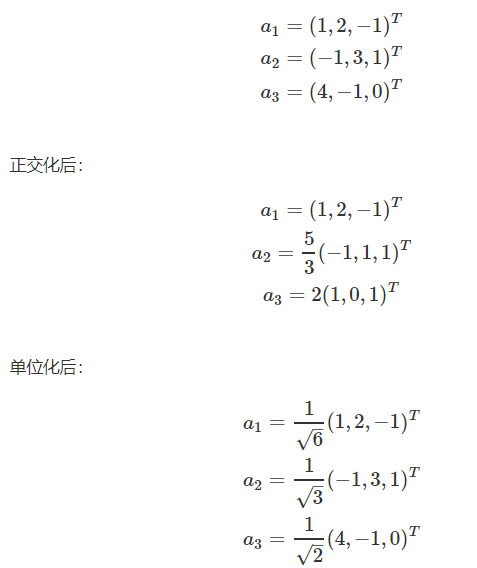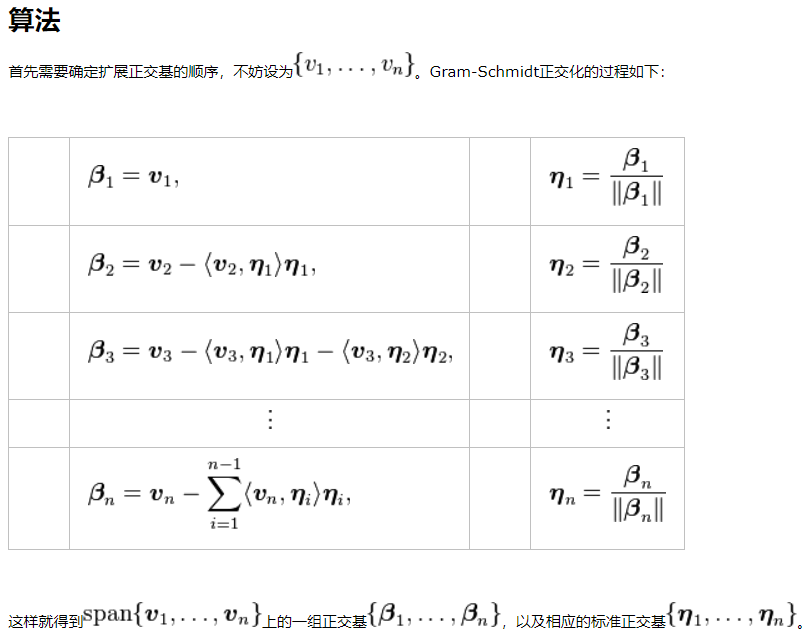求解特征值和特征向量

一、特征值和特征向量的概念和计算
明确定义：设A是n阶方阵，如果存在常数及非零n向量x，使得，则称是矩阵A的特征值，x是A属于特征值的特征向量。给定n阶矩阵A，行列式的结果是关于的一个多项式，成为矩阵A的特征多项式，该特征多项式构成的方程称为矩阵A的特征方程。

定理：n阶矩阵A的n个特征值就是其特征方程的n个跟；而A的属于特征值的特征向量就是齐次线性方程的非零解。

齐次线性方程：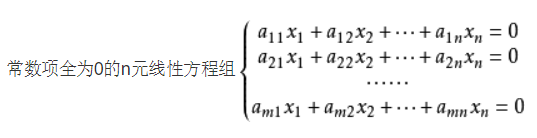例：求的特征值和特征向量
解：，解一元二次方程可得，；对应的特征向量为x满足，求得对应的特征向量为x满足，求得二、特征值和特征向量的几何意义
1、矩阵、向量、向量的矩阵变换
在进行特征和特征向量的几何意义解释之前，我们先回顾一下向量、矩阵、向量矩阵变换的等相关知识。
向量有行向量和列向量，向量在几何上被解释成一系列与轴平行的位移，一般说来，任意向量v都能写成"扩展"形式：以3维向量为例，定义p、q、r为指向+x，+y和+z方向的单位向量，则有v=xp+yq+zr。现在向量v就被表示成p、q、r的线性变换了。这里的基向量是笛卡尔积坐标轴，但事实上这个一个坐标系可以由任意的3个基向量定义，只要这3个基向量线性无关就行（不在同一平面上）。因此，用一个矩阵乘以向量，如Ax，表述如下：如果把矩阵的行解释为坐标系的基向量，矩阵与向量相乘（或向量与矩阵相乘）相当于执行一次坐标转换，Ax=y可表述为x经矩阵A变换后变为y。因此，追溯矩阵的由来，与向量的关系，我们会觉得矩阵并不神秘，它只是用一种紧凑的方式来表达坐标转换所需的数学运算。
2、矩阵的特征值和特征向量
矩阵A的特征值和特征向量分别为和x，记为，该式子可理解为向量x在几何空间中经过矩阵A的变换后得到向量。由此可知，向量x经过矩阵A变换后，方向并无改变（反方向不算方向改变），只是伸缩了倍。
以矩阵为例，其特征向值分别为，，对应的特征向量为，，那么（）表示向量经过矩阵A变换后，得到，向量变换变为改变方向，知识将在原方向上扩充了2倍。特征值也是同样道理，经过矩阵A变换后特征向量在原方向上扩充了3倍。
因此，将特征向量看成基向量，矩阵就是这些基向量向对应的特征值伸展所需的数学运算。给定一个矩阵，就可以找出对应的基（特征向量），及透过向量变换（矩阵），这些基的伸展（特征值）。
三、特征值和特征向量的应用实例
1、主成分分析（Principle Component Analysis, PCA）
（1）方差、协方差、相关系数、协方差矩阵
方差：协方差：,,**方差是衡量单变量的离散程度，协方差是衡量两个变量的相关程度（亲疏），协方差越大表明两个变量越相似（亲密），协方差越小表明两个变量之间相互独立的程度越大。
相关系数：，**协方差和相关系数都可以衡量两个表明的相关程度，协方差未消除量纲，不同变量之间的协方差大小不能直接比较，而相关系数消除了量纲，可以比较不同变量之间的相关程度。
协方差矩阵：如果有两个变量X，Y，那么协方差矩阵为，协方差阵说明了样本中变量间的亲疏关系。
（2）主成分分析的思想和算法
主成分分析是利用降维的思想，将多个变量转化为少数几个综合变量（即主成分），其中每个主成分都是原始变量的线性组合，各主成分之间互不相关，从而这些主成分能够反映始变量的绝大部分信息，且所含的信息互不重叠。它是一个线性变换，这个变换把数据变换到一个新的坐标系统中，使得任何数据投影的第一大方差在第一个坐标(称为第一主成分)上，第二大方差在第二个坐标(第二主成分)上，依次类推。主成分分析经常用减少数据集的维数，同时保持数据集的对方差贡献最大的特征。
假设用p个变量来描述研究对象，分别用X1，X2…Xp来表示，这p个变量构成的p维随机向量为X=(X1，X2…Xp)，n个样本构成组成了n行p列的矩阵A。主成分求解过程如下：
第一步，求解得到矩阵A的协方差阵B；
第二步，求解协方差阵B，得到按大小顺序排列的特征值向量，为特征值向量中每个特征值组成的对角矩阵，U为所有特征值对应的特征向量构成的矩阵U，因此有。重点来了，U是有特征向量构成的正定阵，向量的每一行可以视为一个的基向量，这些基向量经过矩阵B转换后，得到了在各个基向量上的伸缩，伸缩的大小即为特征向量。
第三步，主成分个数选择，根据特征值的大小，将特征值较大的作为主成分，其对应的特征向量就为基向量，特征值的筛选根据实际情况而定，一般大于1即可考虑作为主成分。
（3）实例分析——机器学习中的分类问题
机器学习中的分类问题，给出178个葡萄酒样本，每个样本含有13个参数，比如酒精度、酸度、镁含量等，这些样本属于3个不同种类的葡萄酒。任务是提取3种葡萄酒的特征，以便下一次给出一个新的葡萄酒样本的时候，能根据已有数据判断出新样本是哪一种葡萄酒。
问题详细描述：http://archive.ics.uci.edu/ml/datasets/Wine训练样本数据：http://archive.ics.uci.edu/ml/machine-learning-databases/wine/wine.data
把数据集赋给一个178行13列的矩阵R，它的协方差矩阵C是13行13列的矩阵，对C进行特征分解，对角化，其中U是特征向量组成的矩阵，D是特征之组成的对角矩阵，并按由大到小排列。然后，令，就实现了数据集在特征向量这组正交基上的投影。嗯，重点来了，中的数据列是按照对应特征值的大小排列的，后面的列对应小特征值，去掉以后对整个数据集的影响比较小。比如，现在我们直接去掉后面的7列，只保留前6列，就完成了降维。
下面我们看一下降维前和降维后的使用svm分类结果，本部分采用实现SVM的R语言包e1071，代码如下表所示。分类结果显示，使用主成分分析后的样本和未进行主成分分析样本的分类结果一样。因此，主成分分析提取的6个主成分能较好的表达原样本的13个变量。

library("e1071")
#读取数据

#计算协方差阵
covariance = cov(wineData[2:14])

#计算特征值和特征向量
eigenResult=eigen(covariance)

#选取6个主成分,并计算这6个主成分解释的方差总和
PC_NUM = 6
varSum=sum(eigenResult$values[1:PC_NUM])/sum(eigenResult$values)

#降维后的样本
ruduceData= data.matrix(wineData[2:14])%*%eigenResult$vectors[,1:PC_NUM] #加入分类标签 #finalData=cbind(wineData$class,ruduceData)

#给finalData添加列名
#colnames(finalDat) =c("calss","pc1","pc2","pc3","pc4","pc5","pc6")

#训练样本--主成分分析后的样本作为训练样本
y=wineData\$class;
x1=ruduceData;
model1 <- svm(x1, y,cross=10)
pred1 <- predict(model1, x1)
#pred1 <- fitted(model1)
table(pred1, y) #使用table来查看预测结果

#训练样本--原数据作为训练样本
x2=wineData[2:14]
model2 <- svm(x2, y,cross=10)
#pred2 <- predict(model2, x2)
pred2 <- fitted(model2)
table(pred2, y) #使用table来查看预测结果

转载于:https://www.cnblogs.com/shona/p/10716442.html
展开全文• GNN特征分解 概述 这是我们工作的存储库：GNN特征分解，被RTAS 2021（Brif行业跟踪）接受，名为“优化边缘计算平台上的图形神经网络的内存效率” 图神经网络（GNN）在各种工业任务上都达到了最先进的性能。 但是，...Python
• 针对直扩信号伪随机序列（PN码）的估计问题，在基于协方差矩阵特征分解算法的基础上，利用最大范数准则加以改进，提出了基于最大范数准则与矩阵特征分解的伪码序列估计方法，并对其进行了理论分析和实验仿真。...
• 用于信号分解，基于Python语言实现，学习交流
• Efficient PyTorch Hessian eigendecomposition using the Hessian-vector product and stochastic power iteration
• 此提交包含用于通过基于频谱分而治之的高效稳定算法计算对称矩阵 (QDWHEIG.M) 的特征分解和奇异值分解 (QDWHSVD.M) 的函数。 计算结果往往比 MATLAB 的内置函数 EIG.M 和 SVD.M 给出的结果更准确。 函数 TEST.M ...matlab
• 矩阵的特征分解在功率谱估计中的应用，适合通信、电子等专业学习
• 分类目录：《算法设计与分析》总目录 许多数学对象可以通过将它们分解成多个组成部分或者找到它们的一些属性而更好地理解，这些属性是通用的，而不是由我们选择表示它们的方式产生的。...特征分解是使用最广的
分类目录：《算法设计与分析》总目录

许多数学对象可以通过将它们分解成多个组成部分或者找到它们的一些属性而更好地理解，这些属性是通用的，而不是由我们选择表示它们的方式产生的。例如，整数可以分解为质因数。我们可以用十进制或二进制等不同方式表示整数12，但是12=2×2×3永远是对的。从这个表示中我们可以获得一些有用的信息，比如12不能被5整除，或者12的倍数可以被3整除。
正如我们可以通过分解质因数来发现整数的一些内在性质，我们也可以通过分解矩阵来发现矩阵表示成数组元素时不明显的函数性质。特征分解是使用最广的矩阵分解之一，即我们将矩阵分解成一组特征向量和特征值。
方阵

A

A

的特征向量是指与

A

A

相乘后相当于对该向量进行缩放的非零向量

v

v

：

A

v

=

λ

v

Av=\lambda v

标量

λ

\lambda

被称为这个特征向量对应的特征值。类似地，我们也可以定义左特征向量

v

T

A

=

λ

v

v^TA=\lambda v

，但是通常我们更关注右特征向量
如果

v

v

是

A

A

的特征向量，那么任何缩放后的向量

s

v

(

s

∈

R

,

s

≠

0

)

sv(s\in R, s\neq 0)

也是

A

A

的特征向量。此外，

s

v

sv

和

v

v

有相同的特征值。基于这个原因，通常我们只考虑单位特征向量。
假设矩阵

A

A

有

n

n

个线性无关的特征向量

{

v

(

1

)

,

v

(

2

)

,

⋯

,

v

(

n

)

}

\{v^{(1)}, v^{(2)},\cdots, v^{(n)}\}

，对应着特征值

{

λ

i

,

λ

2

,

⋯

,

λ

n

}

\{\lambda_i, \lambda_2, \cdots, \lambda_n\}

。我们将特征向量连接成一个矩阵，使得每一列是一个特征向量：

V

=

[

v

(

1

)

,

v

(

2

)

,

⋯

,

v

(

n

)

]

V=[v^{(1)}, v^{(2)},\cdots, v^{(n)}]

类似地，我们也可以将特征值连接成一个向量：

λ

=

[

λ

i

,

λ

2

,

⋯

,

λ

n

]

T

\lambda=[\lambda_i, \lambda_2, \cdots, \lambda_n]^T

此时，

A

A

的 特征分解可以记作：

A

=

V

diag

(

λ

)

V

−

1

A=V\text{diag}(\lambda)V^{-1}

我们已经看到了构建具有特定特征值和特征向量的矩阵，能够使我们在目标方向上延伸空间。然而，我们也常常希望将矩阵分解成特征值和特征向量。这样可以帮助我们分析矩阵的特定性质，就像质因数分解有助于我们理解整数。
不是每一个矩阵都可以分解成特征值和特征向量。在某些情况下，特征分解存在，但是会涉及复数而非实数。幸运的是，我们通常只需要分解一类有简单分解的矩阵。具体来讲，每个实对称矩阵都可以分解成实特征向量和实特征值：

A

=

Q

Λ

Q

T

A=Q\Lambda Q^T

其中

Q

Q

是

A

A

的特征向量组成的正交矩阵，

Λ

\Lambda

是对角矩阵。特征值

Λ

i

,

i

\Lambda_{i, i}

对应的特征向量是矩阵

Q

Q

的第

i

i

列，记作

Q

:

,

i

Q_{:, i}

。因为

Q

Q

是正交矩阵，我们可以将

A

A

看作沿方向

v

(

i

)

v^{(i)}

延展

λ

i

\lambda_i

倍的空间。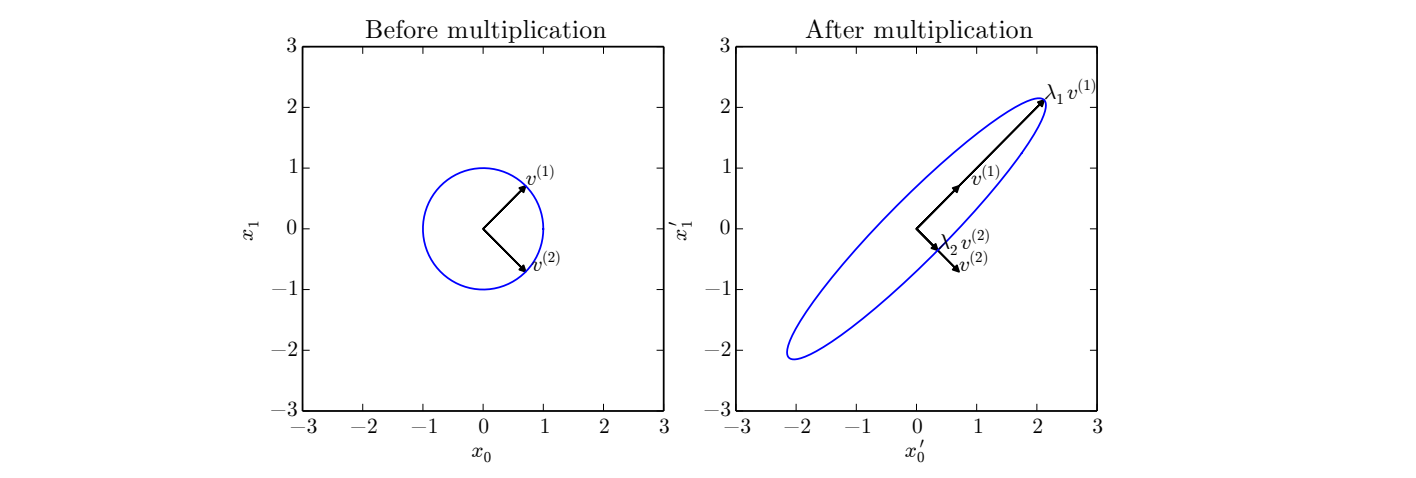虽然任意一个实对称矩阵

A

A

都有特征分解，但是特征分解可能并不唯一。如果两个或多个特征向量拥有相同的特征值，那么在由这些特征向量产生的生成子空间中，任意一组正交向量都是该特征值对应的特征向量。因此，我们可以等价地从这些特征向量中构成

Q

Q

作为替代。按照惯例，我们通常按降序排列

Λ

\Lambda

的元素。在该约定下，特征分解唯一当且仅当所有的特征值都是唯一的。
矩阵的特征分解给了我们很多关于矩阵的有用信息。矩阵是奇异的当且仅当含有零特征值。实对称矩阵的特征分解也可以用于优化二次方程

f

(

x

)

=

x

T

A

x

f(x)=x^TAx

，其中限制

∣

∣

x

∣

∣

2

=

1

||x||_2=1

。当

x

x

等于

A

A

的某个特征向量时，

f

f

将返回对应的特征值。在限制条件下，函数∫的最大值是最大特征值，最小值是最小特征值。
所有特征值都是正数的矩阵被称为正定；所有特征值都是非负数的矩阵被称为半正定。同样地，所有特征值都是负数的矩阵被称为负定；所有特征值都是非正数的矩阵被称为半负定。半正定矩阵受到关注是因为它们保证：

∀

x

:

x

T

A

x

≥

0

\forall x:x^TAx\geq 0

此外，正定矩阵还保证：

x

T

A

x

=

0

⇒

x

=

0

x^TAx=0\Rightarrow x=0


展开全文机器学习 深度学习 数学 线性代数
• 只可以用在方阵上2.1.1 特征分解的原理2.1.2 特征分解的合理性2.1.3 特征分解的计算2.1.4 对称矩阵的特征分解（这个性质后面SVD推导用到） 1. 前言 要学会矩阵的特征分解，可以提前看矩阵的一些基础知识： ...


文章目录
1. 前言2.矩阵的进阶知识2.1 特征分解（谱分解）=>只可以用在方阵上2.1.1 特征分解的原理2.1.2 特征分解的合理性2.1.3 特征分解的计算2.1.4 对称矩阵的特征分解（这个性质后面SVD推导用到）

1. 前言
要学会矩阵的特征分解，可以提前看矩阵的一些基础知识： https://blog.csdn.net/qq_30232405/article/details/104588293
2.矩阵的进阶知识
2.1 特征分解（谱分解）=>只可以用在方阵上
2.1.1 特征分解的原理
如果说一个向量

v

v

是方阵

A

A

的特征向量，将一定可以表示成下面的形式：

A

v

=

λ

v

(2-1)

Av=\lambda v \tag{2-1}

这种形式在数学上的含义：描述的是矩阵

A

A

对向量

v

v

的变换效果只有拉伸，没有旋转。(因为

λ

\lambda

这个值是一个数值)这时候

λ

\lambda

就被称为特征向量

v

v

对应的特征值
也可以看成矩阵

A

A

，向量

v

v

，系数

λ

\lambda

这三者建立了一种联系，但显然我们无法通过式(2-1)来用

v

v

和

λ

\lambda

表示

A

A

，因为这个式子不是完备的，对于一个秩为

m

m

的矩阵

A

A

，应该存在

m

m

个这样的式子，完备式子应该是：

A

(

v

1

,

v

2

,

.

.

.

,

v

m

)

=

(

λ

1

v

1

,

λ

2

v

2

,

.

.

.

,

λ

m

v

m

)

=

(

v

1

,

v

2

,

.

.

.

,

v

m

)

[

λ

1

.

.

.

0

⋮

⋱

⋮

0

.

.

.

λ

m

]

A(v_1,v_2,...,v_m)=(\lambda_1 v_1,\lambda_2 v_2,...,\lambda_m v_m)=(v_1,v_2,...,v_m) \begin{bmatrix} \lambda_1 & ... & 0 \\ \vdots & \ddots & \vdots \\ 0 & ... & \lambda_m \\ \end{bmatrix}

A

V

=

V

Λ

(2-2)

AV=V\Lambda \tag{2-2}

根据公式(2-2)就可以得到矩阵

A

A

的特征分解公式：

A

=

V

Λ

V

−

1

(2-3)

A=V\Lambda V^{-1} \tag{2-3}

矩阵的一组特征向量

V

V

是一组正交向量。其中

V

V

是这个矩阵

A

A

的特征向量组成的矩阵，

Λ

\Lambda

是一个对角阵，每一个对角线上的元素就是一个特征值。
总结：特征分解，可以得到

m

m

个特征向量和特征值，利用这

m

m

个特征（代表这个矩阵最重要的特征），就可以近似这个矩阵。
2.1.2 特征分解的合理性
一个矩阵和该矩阵的非特征向量相乘是对该向量的旋转变换；一个矩阵和该矩阵的特征向量相乘是对该向量的伸缩变换，其中伸缩程度取决于特征值大小。
矩阵在特征向量所指的方向上具有 增强（或减弱）特征向量 的作用。这也就是说，如果矩阵持续地叠代作用于向量，那么特征向量的就会突显出来，利用python进行计算：
首先举一个例子，假设矩阵

A

A

和向量

V

V

:

A

=

[

4

1

1

1

2

1

3

2

3

]

A= \begin{bmatrix} 4 & 1 & 1 \\ 1 & 2 & 1 \\ 3 & 2 & 3 \\ \end{bmatrix}

V

=

[

−

1

5

3

]

V = \begin{bmatrix} -1 \\ 5 \\ 3 \\ \end{bmatrix}

用矩阵

A

A

去反复左乘一个向量

V

V

，python代码如下：
import numpy as np
import copy
A = np.array([[4, 1, 1], [1, 2, 1], [3, 2, 3]])
V = np.array([[-1], , ])
dot_nums = [1, 3, 5, 10]
for i in range(len(dot_nums)):
A_ = copy.copy(A)
for _ in range(dot_nums[i] - 1):
A_ = np.dot(A_, A)
B = np.dot(A_, V)
B = np.abs(B)
C = B / np.sum(B)
print("dot number: %d" % dot_nums[i])
print(C)

得到结果：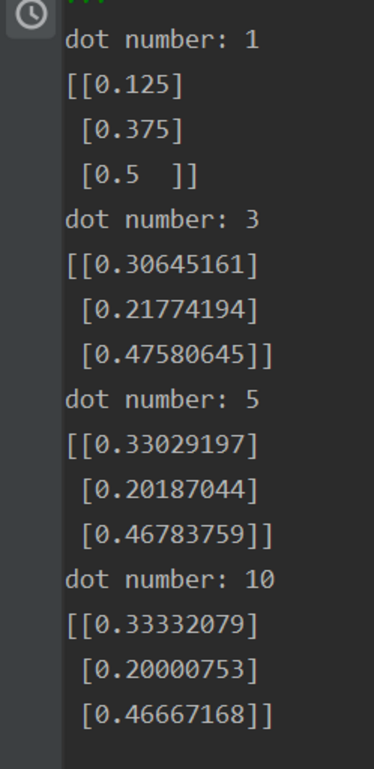可以看到不断左乘A后，变换后的归一化向量在（0.33，0.2，0.46）附近徘徊，这与计算出来的最大特征值对应的特征向量归一化后的结果是一致的，这也就佐证了矩阵是具有某种不变的特性的。因此为了提取矩阵这种“不变性”，或者说是为了描述变换（矩阵惩罚是一种线性变换）的主要方向是非常有必要的。
2.1.3 特征分解的计算
在 (2-1) 式的基础上，进行一些变形 ：

A

v

=

λ

v

→

A

v

=

λ

I

v

→

(

λ

I

−

A

)

v

=

0

(2-4)

Av=\lambda v \to Av=\lambda Iv \to (\lambda I-A)v = 0 \tag{2-4}

根据线性方程组理论，为了使这个方程有非零解，矩阵

(

λ

I

−

A

)

(\lambda I-A)

的行列式必须是零:

d

e

t

(

λ

I

−

A

)

v

=

0

(2-5)

det(\lambda I-A)v = 0 \tag{2-5}

上式也被称为是

A

A

的特征方程，计算出所有

λ

\lambda

的取值后，再代入

(

λ

I

−

A

)

v

=

0

(\lambda I-A)v = 0

求解对应的

v

v

注意：要注意特征值是重根时的情况。。。。
（1）手算
求矩阵

A

A

的特征值和特征向量：

∣

λ

I

−

A

∣

=

∣

4

1

1

1

2

1

3

2

3

∣

=

(

λ

−

6

)

(

λ

−

2

)

(

λ

−

1

)

|\lambda I-A| = \begin{vmatrix} 4 & 1 & 1 \\ 1 & 2 & 1 \\ 3 & 2 & 3 \\ \end{vmatrix} = (\lambda-6)(\lambda-2)(\lambda-1)

可以得到结果：

λ

1

=

6

,

λ

2

=

2

,

λ

3

=

1

\lambda_1 = 6, \lambda_2 = 2, \lambda_3=1

当

λ

=

6

\lambda=6

时，

(

6

I

−

A

)

v

=

0

(6I-A)v=0

:

(

4

1

1

1

2

1

3

2

3

)

(

v

1

v

2

v

3

)

=

0

\begin{pmatrix} 4 & 1 & 1 \\ 1 & 2 & 1 \\ 3 & 2 & 3 \\ \end{pmatrix} \begin{pmatrix} v_1 \\ v_2 \\ v_3 \\ \end{pmatrix} = 0

r

e

s

u

l

t

:

v

1

=

5

,

v

2

=

3

,

v

3

=

7

result: v_1 = 5, v_2=3, v_3=7

当

λ

=

2

\lambda=2

时，

(

2

I

−

A

)

v

=

0

(2I-A)v=0

:

r

e

s

u

l

t

:

v

1

=

1

,

v

2

=

−

1

,

v

3

=

1

result: v_1 = 1, v_2=-1, v_3=1

当

λ

=

1

\lambda=1

时，

(

I

−

A

)

v

=

0

(I-A)v=0

:

r

e

s

u

l

t

:

v

1

=

0

,

v

2

=

1

,

v

3

=

−

1

result: v_1 = 0, v_2=1, v_3=-1

（2）python计算
使用python中自带的库eig，其中

V

V

为特征向量矩阵，

D

D

为特征值。

V

V

中的列是对应的每一个特征向量
import numpy as np
import copy
A = np.array([[4, 1, 1], [1, 2, 1], [3, 2, 3]])
D, V = np.linalg.eig(A)
if np.equal(np.dot(A, V), np.dot(V, np.diag(D))):
print(True)

结果为：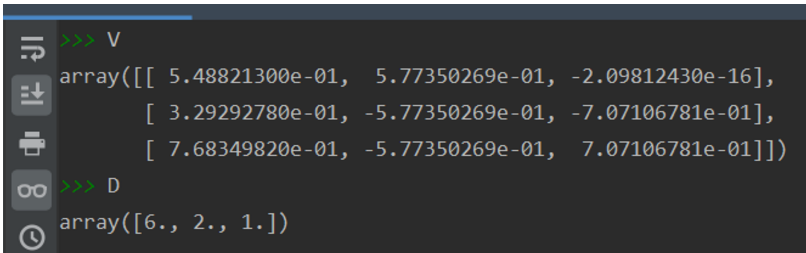发现python计算的和手算的特征向量值不同，但比例是一样的，这是因为特征向量不是唯一的，特征向量来自齐次线性方程组的解，是齐次线性方程组的基础解系的非零线性组合。
2.1.4 对称矩阵的特征分解（这个性质后面SVD推导用到）
定理：假设矩阵

A

A

是一个对称矩阵，则其不同特征值对应的特征向量两两正交。
证明：
首先进行特征分解：

A

x

i

=

λ

i

x

i

(2-6)

Ax_i=\lambda_i x_i \tag{2-6}

A

x

j

=

λ

j

x

j

(2-7)

Ax_j=\lambda_j x_j \tag{2-7}

在公式(2-6)左乘

x

j

x_j

:

x

j

T

A

x

i

=

λ

i

x

j

T

x

i

(2-8)

x_j^\mathrm{T} Ax_i=\lambda_i x_j^\mathrm{T} x_i \tag{2-8}

因为矩阵A是一个对称矩阵，可以对式(2-8)的左边做如下变换：

x

j

T

A

x

i

=

x

j

T

A

T

x

i

=

(

A

x

j

)

T

x

i

=

(

λ

j

x

j

)

T

x

i

=

λ

i

x

j

T

x

i

(2-9)

x_j^\mathrm{T} Ax_i=x_j^\mathrm{T} A^\mathrm{T} x_i = (Ax_j)^\mathrm{T} x_i = (\lambda_j x_j)^\mathrm{T}x_i = \lambda_i x_j^\mathrm{T} x_i \tag{2-9}

最后通过(2-9)可以得到：

(

λ

j

x

j

)

T

x

i

=

λ

i

x

j

T

x

i

→

(

λ

j

−

λ

i

)

x

j

T

x

i

=

0

(2-10)

(\lambda_j x_j)^\mathrm{T}x_i = \lambda_i x_j^\mathrm{T} x_i \to (\lambda_j - \lambda_i)x_j^\mathrm{T}x_i = 0 \tag{2-10}

因为

λ

j

≠

λ

i

\lambda_j \neq \lambda_i

，

x

j

T

x

i

x_j^\mathrm{T}x_i

必然等于0。 由于

x

j

x_j

和

x

i

x_i

是矩阵A的任意两个特征向量，所以命题得证。
展开全文线性代数 python 机器学习 算法 矩阵
• 这篇文章的主要内容包括：1、什么是特征分解2、什么是奇异值分解3、如何求解特征值和特征向量4、特征值和特征向量有什么意义一、特征分解特征分解(eigendecomposition)：是使用最广的矩阵分解之一，通过特征分解可以...
特征分解和奇异值分解在机器学习的应用中经常出现，在学习线性代数的时候也学习过。线性代数学完之后，之后去按照步骤去求解特征值和特征向量，也没搞明白特征值和特征向量究竟有什么作用。这篇文章的主要内容包括：
1、什么是特征分解
2、什么是奇异值分解
3、如何求解特征值和特征向量
4、特征值和特征向量有什么意义
一、特征分解
特征分解(eigendecomposition)：是使用最广的矩阵分解之一，通过特征分解可以将矩阵分解成一组特征值和特征向量。方阵A的特征向量(eigenvector)是指与A相乘后相当于对该向量进行缩放的非零向量v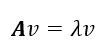其中v就是方阵A的特征向量，λ就是A的特征值。如果v是A的特征向量，那么任何缩放后的向量s*v(s为任意实数，且不为0)也是
展开全文奇异值分解 特征向量 特征值
• 特征分解（Eigendecomposition），又称谱分解（Spectral decomposition）是将矩阵分解为由其特征值和特征向量表示的矩阵之积的方法。 需要注意只有对可对角化矩阵才可以施以特征分解。 令A是一个 N×N 的方阵，且...
• 一、特征分解（特征值、特征向量） 二、正定、半正定、负定 三、奇异值（SVD）分解 一、特征分解（特征值、特征向量） 许多数学对象可以通过将它们分解成多个组成部分或者找到它们的一些属性以便更好地理解，...奇异值分解 SVD
• Eigen库为是一个矩阵运算的库，实现Matlab仿真中的各种矩阵运算，我用过特征分解eig，奇异值分解SVD，对角阵，行，列最大，等等。Matlab可以实现的这里几乎都有，使用方法见本博客中的介绍
• 5.2.4 方阵的特征分解 5.2.4.1 特征值、特征向量 A x = λ x A x = λ x Ax=\lambda x 的几何意义： A x A x Ax 是指对向量x进行了旋转。当x为A的特征向量时，旋转后的向量与原向量共线，其缩放倍数为...
• ## 特征分解条件

千次阅读 2017-07-31 18:21:56
• 线性调频（LFM）信号是高分辨率雷达的主要信号形式之一，通过分析LFM雷达信号实现距离高分辨率成像的原理，针对杂波和噪声对雷达回波信号的影响，提出了利用基于特征分解功率谱估计的方法进行频率估计，从而实现LFM...
• 特征分解于奇异值分解的几何解释 在计算机图形学一中介绍了一些具体的变换矩阵，如旋转，缩放，位移等等，但这些矩阵都有特定的形式，那么如何去理解一个任意的2维或3维变换矩阵的几何意义呢，在本节中将会利用特征...
• 论文——基于特征分解的GFDM实现无ICI、ISI干扰和导频插入用于信道估计的仿真代码
• 非负矩阵分解（NMF）是一种流行的数据分析方法，其目标是使其接近通过所有非负成分产生的两个非负矩阵。文中描述了一种对于多因式非负矩阵分解（mfNMF）问题新的且有效的算法，概括了原始NMF问题的一些因式。此外，...
• 颜庆津版数值分析编程作业，C语言(少量C++语法)实现矩阵的QR分解法迭代求矩阵的全部复数格式特征值，先对矩阵进行拟上三角化再迭代，迭代求出全部特征值后使用列主元素高斯消元法求出所有实特征值对应的特征向量。
• 特征分解——>奇异值分解（SVD）——>隐语义模型（LFM），三个算法在前者的基础上推导而成，按顺序先后出现。三者均用于矩阵降维。其中： 特征分解可用于主成分分析。 奇异值分解（SVD）和隐语义模型（LFM...奇异值分解 隐语义模型 SVD LFM
• 利用MUSIC算法实现信号到达角估计。并分析不同信噪比情况下，性能的变化。
• ## 矩阵的特征分解

万次阅读 多人点赞 2019-01-25 17:42:18
特征分解是使用最广的矩阵分解之一，即我们将矩阵分解为一组特征向量和特征值。 设A为n阶矩阵，若存在常数λ及n维非零向量x，使得Ax=λx，则称λ是矩阵A的特征值，x是A属于特征值λ的特征向量。 如果x是A的特征...
• 特征分解到协方差矩阵：详细剖析和实现PCA算法 本文先简要明了地介绍了特征向量和其与矩阵的关系，然后再以其为基础解释协方差矩阵和主成分分析法的基本概念，最后我们结合协方差矩阵和主成分分析法实现数据降维...PCA 协方差矩阵 基变换
• 3.1 能否进行特征分解 可以特征分解要求 AAA 必须是一个可对角化的矩阵, 满足以下任一条件即可 AAA 可逆 nnn 个线性无关的特征向量/ nnn 个不同的特征值 所有特征值的代数重数 = 几何重数 实对称矩阵 4 对称对角化 S...线性代数 矩阵
• 四、总结 总之，本文的工作将特征融合任务拆分成为特征分解任务，然后对于不同的特征分解做分步融合。本模型有效的利用了先验信息，即base信息代表着大尺度的背景信息， detail信息是互异性较明显的信息。先验信息...算法 人工智能 计算机视觉 深度学习 机器学习
•研究论文...